NKCSD: Unit 6 Math Pre-Test
starstarstarstarstarstarstarstarstarstar
by Rachael Michael
| 13 Questions
1
1 pt
What is one possible way to arrange unit squares to form a rectangle with an area of 54 square units?
A 5 columns of 4 unit squares each
B 6 columns of 8 unit squares each
C 6 columns of 9 unit squares each
D 9 columns of 5 unit squares each2
1 pt
In each shape above, each small square represents 1 square centimeter. Which shape has an area that can be found using the equation above?
W
X
Y
Z3
1 pt
Which of the following is true?
A The rectangles have different area and perimeters.
B The rectangles have the same area and perimeters.
C The rectangles have the same area, but different perimeters.
D The rectangles have the same perimeter, but different areas.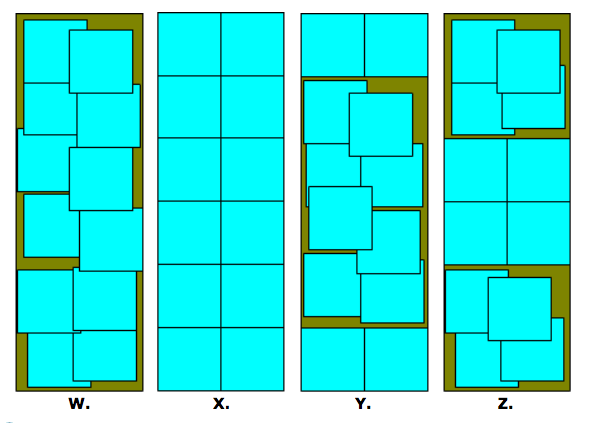4
1 pt
Which figure above shows the correct way to cover the piece of paper with unit squares to show an area of 12 square units?
W
X
Y
Z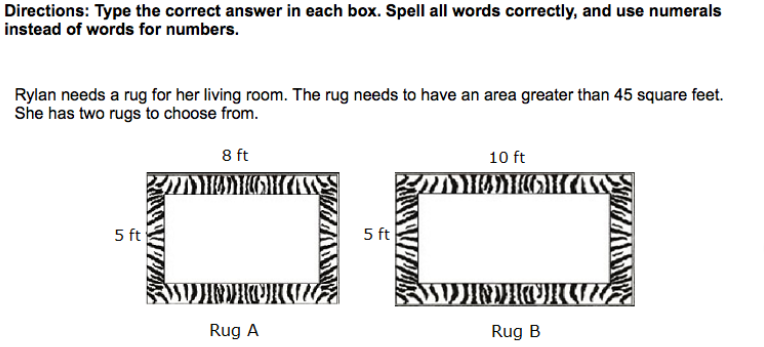5
1 pt
Rug A has an area of ___ square feet.
A 13
B 26
C 40
D 58
6
1 pt
Rug B has an area of ___ square feet.
A 30
B 15
C 50
D 51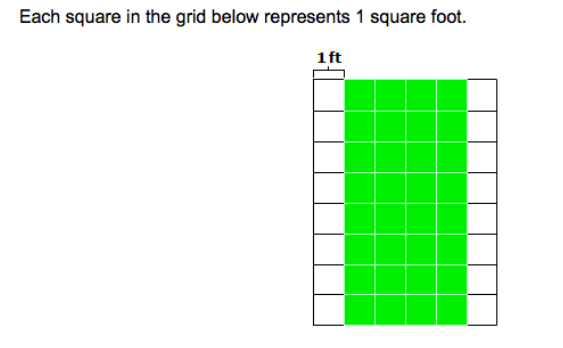7
1 pt
What is the area of the shaded square below?
A 36 square feet
B 28 square feet
C 32 square feet
D 24 square feet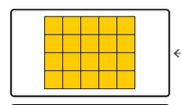8
1 pt
Which equation matches the area of the rectangle above?
A 3 x 7= 21
B 3 x 6=18
C 4 x 4=16
D 4 x 5 =20
9
1 pt
Robbie's kite has two sides that measure 5 inches and two sides that measure 10 inches. What is the perimeter of his kite?
A 30
B 15
C 50
D51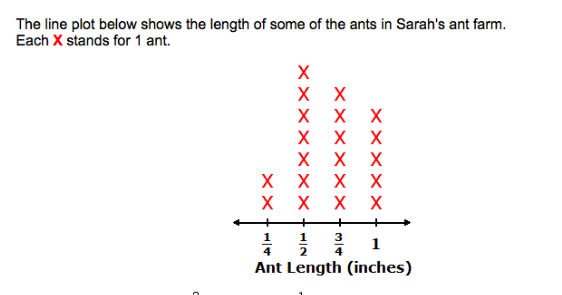10
1 pt
How many more ants were 3/4 inch long than 1/4 inch long?
A 6
B 2
C 4
D 811
1 pt
Which recatngle shows the correct way yo find the total area of the 4 x 5 rectangle?
W
X
Y
Z12
1 pt
A 15 feet
B 16 feet
C 14 feet
D 13 feet
Amber measured different pieces of ribbon. The lengths in inches are shown below. Create a line plot that shows this information. Each X stands for 1 piece of ribbon.
13
12 pts